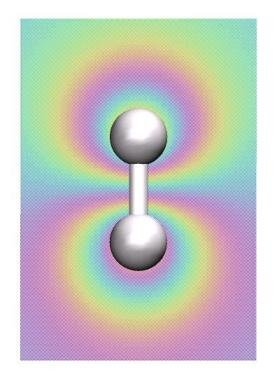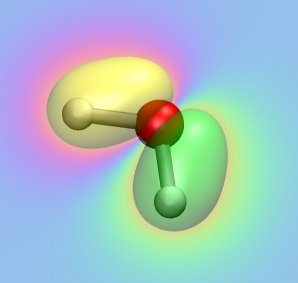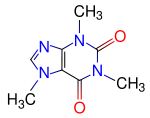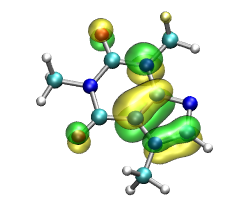# Introduction to Electronic Structure Calculations using MOLPRO

#### Dr. Udo W. Schmitt, Max-Planck Institut fuer Biophysikalische Chemie,GoettingenIn the previous lecture we've learned about the theoretical basis of electronic

structure methods, and in particular about the Hartree-Fock method.

Now it is time to jump into the electronic structure business and perform

a couple of calculations by yourself.

The package we are going to use is called MOLPRO, which has been developed

by Peter Knowles and Hans-Juergen Werner over the last 15 years. It is among

the modern electronic structure codes available and provides an easy-to-use scripting language that allows for

a user-friendly access to quantum chemistry. Additionaly MOLPRO provides for the largest set of implemented post-Hartree-Fock methods.

To learn how to use the program by yourself or maybe extend your knowledge beyond the

scope of this tutorial one can check out the

links "Quick Start" or "User's Manual" on the following site

To start a calculation we basically need four things:

• the structure of the molecule of interest in (cartesian) coordinates

• the overall charge of our system

• the method that will be used (Hartree-Fock (HF),

Density Functional Theory (DFT), Moeller-Plesset perturbation theory (MPx) etc.)

• a basis set.

The files needed for this practical can be downloaded as an archive here

and unpacked by typing


tar xzvf prakt1.tar.gz



### The H-H molecule

Our first calculation will be the simple H2 molecule, which is the smallest

molecule that exhibits all fundamental aspects of chemical bonding (why?).

A simple input for the electronic structure calculation of H2 is given below:


basis=6-31G

r=1.0 ang

geometry={angstrom;

h1;

h2,h1,r}

hf



First, the basis (6-31G) using the so-called Pople notation is specified. G stands for "Gaussian", so a GTO basis with 6 gaussian functions for the inner (non-valence) electrons and 4 (3+1) for

the valence electrons is used. Then, the H-H distance and the so-called internal coordinates (bonds,angles, torsions) are specified.Finally, the key word "hf" stands for "do a Hartree-Fock calculation"

Now we start out first electronic structure calculation by typing


molpro h2.com



During the calculation MOLRPO writes an output file that communicates the most

important infos to the user. Please extract the following information:

• number of SCF cycles needed for convergence

• the HF energy (what units are being used?)

• the dipole moment

Additionaly you could extract the individual HF energies after each SCF cycle and plot them

against the number of cycles.

In the next step we want to perform the visualization of the bonding and antibonding molecular

orbitals (MO) of the H2 molecule. For this we have to redo are electronic structure calculation:


molpro h2_orbitals.com



The same calculation as in the previous step will be performed, but additionaly the MOs

and the electron density are written to external files. To view the MOs, we will have to use VMD, a molecular visualization program for displaying, animating, and analyzing large biomolecular systems using 3-D graphics and built-in scripting.

Typing


vmd -e orbitals.vmd



In the VMD main window you can activate(deactivate) the two MO and the electron density while clicking on the D entry for each MO/density entry in the main window. Which orbital is the bonding, which one is the anti-bonding one?

So far, we have only performed so-called single-point calculations, i.e. computed the HF energy

for a fixed nuclear configuration. MOLPRO is quite versatile when it comes to automatically

perform scans along selected coordinates. In our case of the H2, we will now

determine the energy profile (or the potential energy surface (PES)) along the H-H distance. The input

file now looks like this:


r=[0.3 0.4 0.6 0.8 1.0 1.2 1.4 1.6 2.0 4.0 6.0] ang

basis=6-31G

i=1

geometry={

h1;

h2,h1,r(i)}

do i=1,#r   !loop over range of bond distances

hf

e1(i)=energy

enddo

{table,r,e1

title,HF PES for H-H

plot,file='h2_hf_pes.plot'

}



the program will loop over all specified distances and perform for each of those an independent SCF calculation. Finally, a table of the respective HF energies will be written to a file called h2_hf_pes.plot

Now we going to use the plot program "xmgrace" that comes with most Linux distributions

to look at the PES. Typing


xmgrace h2_hf_pes.plot



will reveal the electronic HF energy as a function of the H-H distance.

What is the energy one would expect for the dissociation limit (i.e. r-> oo) of H-H?

As pointed out in the lecture, the HF method gives molecular interaction energies that are only

approximations to the exact quantum many-body energy. In the following we will study the effect

of improving the HF approximation. This will be achieved via perturbation theory, the so-called

Moeller-Plesset (MPx, where x gives the order in the perturbation expansion) and variational

multi-determinant methods, like e.g. configuration interaction (CI). In molpro this can simply be

achieved by adding the respective keywords:


r=[0.3 0.4 0.6 0.8 1.0 1.2 1.4 1.6 2.0 3.0 4.0 5.0 6.0] ang

basis=6-31G

i=1

geometry={

h1;

h2,h1,r(i)}

do i=1,#r   !loop over range of bond distances

hf          ;e_hf(i)=energy

mp2,NOCHECK ;e_mp2(i)=energy

casscf      ;e_cas(i)=energy

mrci        ;e_ci(i)=energy

enddo

{table,r,e_hf,e_mp2,e_cas,e_ci

title,HF PES for H-H

plot,file='h2_methods_pes.plot'

}After a sucessful finish of the MOLPRO run you can have a look at the four PESs again using "xmgrace".

In our example the CI method (mrci) can be considered as the most accurate. CASSCF stands for "complete active space SCF", which can be regarded as a truncated CI expansion. Compare your result with respect to the obtained equilibrium bonding distance (How large is the error for this quantity?) and the dissociation limit for

each method. Hereby the CI results should serve as the reference energy curve. Repeat the calculation with changing the

order in the perturbation expansion from 2 to 4 (mp2 -> mp4) and compare the two results. What is the error in the CI result

compared to the analytical results of -1 Hartree. How can we improve the CI dissociation energy?

### The water molecule

Now we going to move to a slightly more complex molecule having three nuclei and 10 electrons: the water monomer. For the structural information MOLPRO allows for the use of

the convient xyz in cartesian coordinates format. An example xyz-File is shown here:


3

remark line

O    0.00000   0.00000   0.00000

H    0.00000   0.00000   0.94000

H    0.00000   0.99030  -0.23192



With this structure, which does not represent the well-known equilibrium structure of water, we first have to perform a so-called geometry optimization. During the geometry optimization cycle a SCF calculation is performed. This one is used to compute the gradient of the total energy on the nuclei, which is then used

to perform a move of the nuclei. Then the new nuclei positions are feeded into a new SCF calculation. These

steps are repeated until the structure (=positions of the nuclei) does not change anymore.

The input file for a geometry optimization looks like this:


basis=6-31G                  !basis set

geomtyp=xyz

GEOMETRY=h2o.xyz

hf                           !Hartree-Fock

optg,savexyz=h2o_opt.xyz



Compare the optimized geometry "h2o_opt.xyz" with your input geometry "h2o.xyz" using VMD. Determine the bond distances and the bending angle in the water molecule. How does it compare to the experimental results? How can we further improve the structure? How many geometry optimization cycles were necessary to converge the geometry? (you could also plot the number of cycles against the corresponding HF energy using "xmgrace" or "gnuplot")

Using the optimized geometry, we are now computing the MOs of water.

Have a look at the input file "h2o_orbitals.com" first and try to analyze what is going on

in this type of calculation. Then type


molpro h2o_orbitals.com



The generated MOs can again be visualized using VMD:


vmd -e orbitals.vmd



Have a look at all occupied MOs and elucidate their role for bonding in the water monomer.

Which atomic orbitals of the individual atoms are predominately participating in each MO?

Compare the MOs with the ones shown on the following web site:

Molecular Orbitals of the Water Monomer

### CaffeineFinally we are gonna to compute the electronic structure of the well-known

Caffeine molecule, which is a xanthine alkaloid compound that acts as a stimulant in humans.

The structure of this biomolecule is shown to the left.

Type to start the HF caclulation:


molpro caffeine.com &

tail -f caffeine.out



and observe the information that is written to the screen.

How long does one SCF cycle take on average? How many electrons are involved during this computation?

The so-called HOMO (highest occupied molecular orbital) can be visualized using VMD:


vmd -e orbitals.vmd



How many occpuied MO are there?

If there is some time left, try to compute all orbitals by changing "HOMO" to "ALL" in the input file.

Then visualize them along the same lines as in the previous example.Go to Editor View